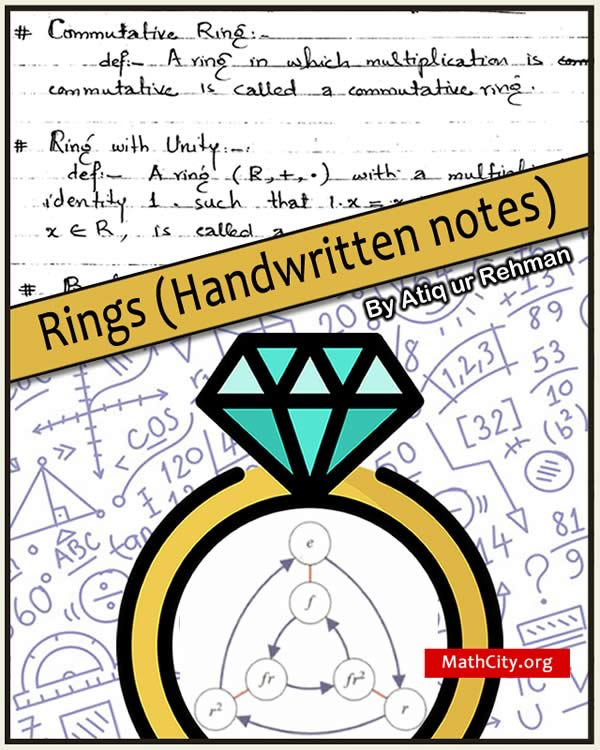# Rings (Handwritten notes)Ring is a two-operation mathematical structure. It is an abelian group with one operation and a semi-group with another operation, and the distributive law is true for the first operation relative to the second operation. This mathematical idea is fundamentally pure. These notes offer a very simple method for learning the concept of rings and other ideas that are closely related to rings. These are lecture notes written by Atiq ur Rehman.

 Name Rings (Handwritten notes)- Lecture Notes Atiq ur Rehman 37 pages PDF (see Software section for PDF Reader) PDF: 3.28 MB
• Rings; definition and examples
• Commutative ring, ring with unity, Boolean's ring, division ring
• Zero divisor and examples, integral domain and related theorems
• Field, examples and related theorems
• Characteristic of ring, examples and related theorems
• Regular ring, examples and related theorems
• Ideals, and related theorem
• Quotient ring
• Homomorphism of a ring, kernel of homomorphism and related theorems
• Principal ideal, principal ideal ring
• Maximal ideal and related theorem
• Fundamental homomorphism theorem

• notes/rings_handwritten_notes